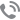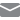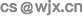# 敬业度报告解读

## 二、报告内分值计算1.计算“敬业度得分”1.先计算每道题的平均分：如：第49题平均分：(4+6+2+8+10+10+6+8)/8=6.75，以此计算，第50题的平均分为：6.75；第51题的平均分为：2.125；第52题的平均分为：7.25

2.敬业度维度得分：该维度下所有题目平均分相加取平均，为（6.75+6.75+2.125+7.25)/4=5.72

“4”表示：4道绑定了敬业度的题目；

2.计算“题目对应的得分”

12题得分：(4+6+2+8+10+10+6+8)/8=6.75

13题得分：(2+8+8+6+10+10+6+4)/8=6.75

14题得分：(2+3+2+2+2+2+2+2)/8=2.125≈2.13

13题得分：(4+8+6+6+10+10+6+8)/8=7.25

3.影响因子

“影响因子”是指，假设统计出的敬业度得分比较低，我们可以查看下影响因子里面的各维度得分，若“企业文化”维度得分较低，我们可以结合企业自身的实际情况分析，是不是因为员工普遍不认同企业文化或工作氛围，所以导致敬业度得分低。统计出"影响因子"的得分，主要是给用户提供分析影响敬业度得分高低的一系类参考指标。1.首先计算每道题的平均分：如：第6题平均分：(6+4+2+6+10+10+4+6)/8=6，以此计算，第7题的平均分为：5.5；第8题的平均分为：8；第9题的平均分为：6

2.然后计算该维度得分：该维度下所有题目平均分相加取平均，为（6+5.5+8+6)/4=6.38

4.敬业度分类比较---END---

•400-993-5858
••广告合作
请扫码添加专员
广告合作